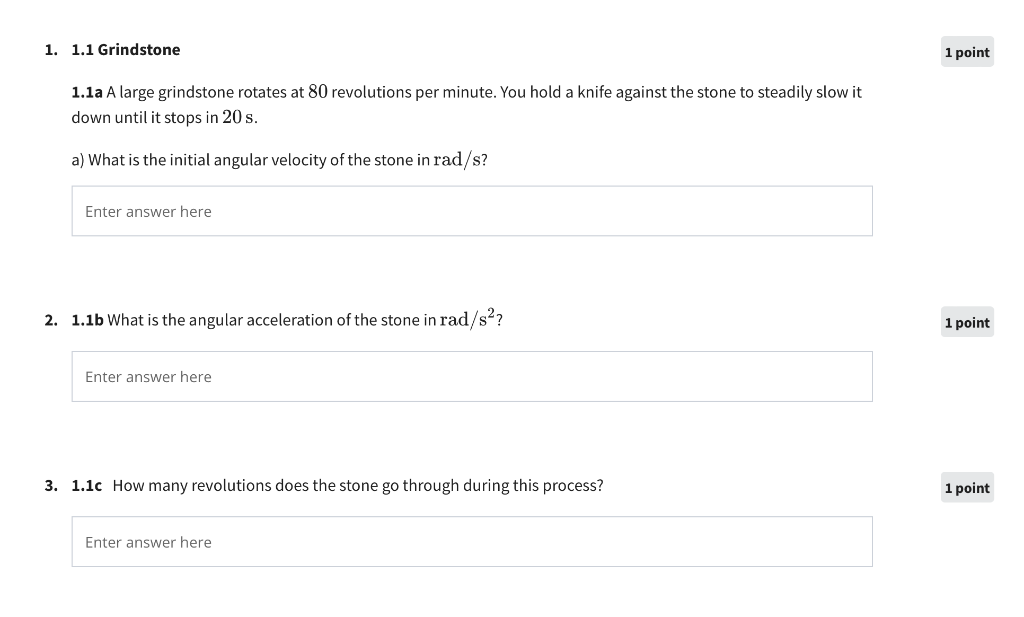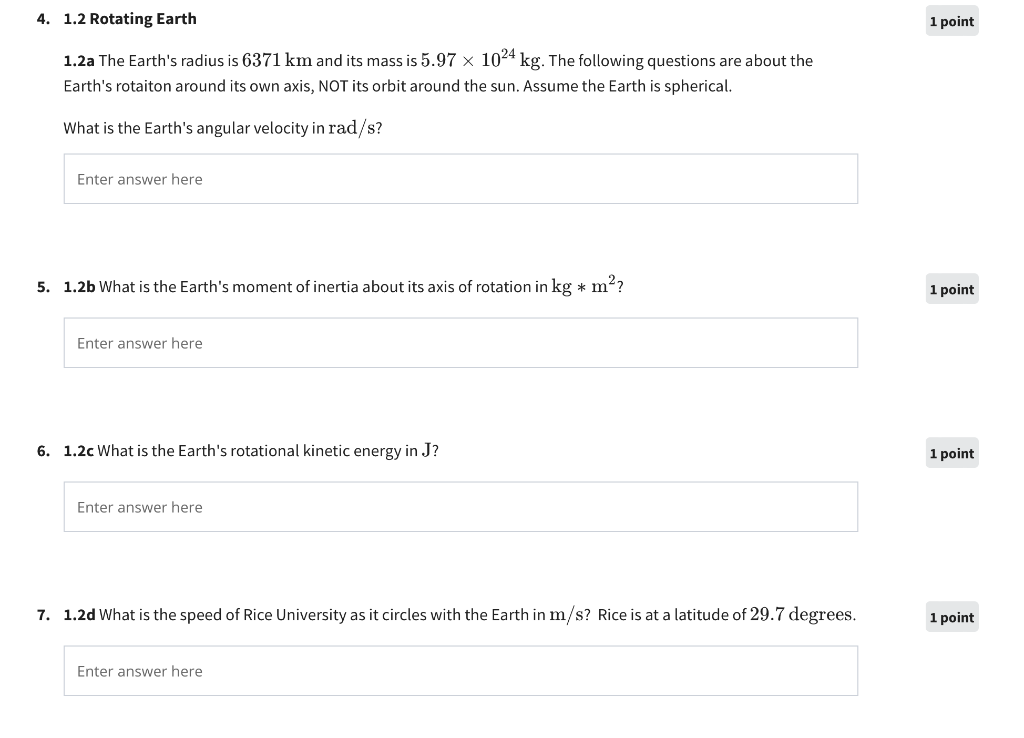# Question ​​​​​​​ 1.1a A large grindstone rotates at 80 revolutions per minute. You hold a knife against the stone to steadily slow it down until it stops in $$20 \mathrm{~s}$$. a) What is the initial angular velocity of the stone in $$\mathrm{rad} / \mathrm{s}$$ ? E 2. 1.1b What is the angular acceleration of the stone in $$\mathrm{rad} / \mathrm{s}^{2}$$ ? 3. 1.1c How many revolutions does the stone go through during this process? 1.2a The Earth's radius is $$6371 \mathrm{~km}$$ and its mass is $$5.97 \times 10^{24} \mathrm{~kg}$$. The following questions are about the Earth's rotaiton around its own axis, NOT its orbit around the sun. Assume the Earth is spherical. What is the Earth's angular velocity in $$\mathrm{rad} / \mathrm{s}$$ ? 5. 1.2b What is the Earth's moment of inertia about its axis of rotation in $$\mathrm{kg} * \mathrm{~m}^{2}$$ ? 6. 1.2c What is the Earth's rotational kinetic energy in $$\mathrm{J}$$ ? 7. 1.2d What is the speed of Rice University as it circles with the Earth in $$\mathrm{m} / \mathrm{s}$$ ? Rice is at a latitude of 29.7 degrees​​​​​​​
Transcribed Image Text: 1.1a A large grindstone rotates at 80 revolutions per minute. You hold a knife against the stone to steadily slow it down until it stops in $$20 \mathrm{~s}$$. a) What is the initial angular velocity of the stone in $$\mathrm{rad} / \mathrm{s}$$ ? E 2. 1.1b What is the angular acceleration of the stone in $$\mathrm{rad} / \mathrm{s}^{2}$$ ? 3. 1.1c How many revolutions does the stone go through during this process? 1.2a The Earth's radius is $$6371 \mathrm{~km}$$ and its mass is $$5.97 \times 10^{24} \mathrm{~kg}$$. The following questions are about the Earth's rotaiton around its own axis, NOT its orbit around the sun. Assume the Earth is spherical. What is the Earth's angular velocity in $$\mathrm{rad} / \mathrm{s}$$ ? 5. 1.2b What is the Earth's moment of inertia about its axis of rotation in $$\mathrm{kg} * \mathrm{~m}^{2}$$ ? 6. 1.2c What is the Earth's rotational kinetic energy in $$\mathrm{J}$$ ? 7. 1.2d What is the speed of Rice University as it circles with the Earth in $$\mathrm{m} / \mathrm{s}$$ ? Rice is at a latitude of 29.7 degrees
Transcribed Image Text: 1.1a A large grindstone rotates at 80 revolutions per minute. You hold a knife against the stone to steadily slow it down until it stops in $$20 \mathrm{~s}$$. a) What is the initial angular velocity of the stone in $$\mathrm{rad} / \mathrm{s}$$ ? E 2. 1.1b What is the angular acceleration of the stone in $$\mathrm{rad} / \mathrm{s}^{2}$$ ? 3. 1.1c How many revolutions does the stone go through during this process? 1.2a The Earth's radius is $$6371 \mathrm{~km}$$ and its mass is $$5.97 \times 10^{24} \mathrm{~kg}$$. The following questions are about the Earth's rotaiton around its own axis, NOT its orbit around the sun. Assume the Earth is spherical. What is the Earth's angular velocity in $$\mathrm{rad} / \mathrm{s}$$ ? 5. 1.2b What is the Earth's moment of inertia about its axis of rotation in $$\mathrm{kg} * \mathrm{~m}^{2}$$ ? 6. 1.2c What is the Earth's rotational kinetic energy in $$\mathrm{J}$$ ? 7. 1.2d What is the speed of Rice University as it circles with the Earth in $$\mathrm{m} / \mathrm{s}$$ ? Rice is at a latitude of 29.7 degrees&#12304;General guidance&#12305;The answer provided below has been developed in a clear step by step manner.Step1/5IntroductionAngular velocity is a measure of the rate of change of angular displacement, which is the angle through which an object rotates or revolves around a fixed axis. It is a vector quantity that describes the speed and direction of rotation of an object, and is measured in radians per second (rad/s) or degrees per second (&#176;/s). Angular velocity is calculated by dividing the change in angular displacement by the time taken for the change to occur, and is used in a variety of applications, including physics, engineering, and astronomy.Angular velocity is the rate of change of angular displacement with respect to time. It is a measure of how fast an object is rotating around a fixed axis. Angular velocity is a vector quantity, meaning that it has both magnitude and direction. It is measured in radians per second (rad/s) or degrees per second (&#176;/s). The formula for angular velocity is:\begin{align*} \mathrm{\omega} &= \mathrm{\Delta\theta\div\Delta{t}} \end{align*}where &#969; is the angular velocity, &#916;&#952; is the change in angular displacement, and &#916;t is the time taken for the change to occur. The direction of the angular velocityis perpendicular to the plane of rotation and follows the right-hand rule. Angular velocity is used in many fields, including physics, engineering, and astronomy.Explanationhe direction of the angular velocityis perpendicular to the plane of rotation and follows the right-hand rule. Angular velocity is used in many fields, including physics, engineering, and astronomy.Explanation:Please refer to solution in this step.Step2/5&#160;a)To find the initial angular velocity of the stone, we can use the formula:\begin{align*} \mathrm{\omega} &= \mathrm{\Delta\theta\div\Delta{t}} \end{align*}where &#969; is the angular velocity in radians per second, &#916;&#952; is the change in angle (in radians), and &#916;t is the time interval.At the start of the process, the grindstone is rotating at 80 revolutions per minute, which is equivalent to:\begin{align*} \mathrm{\omega{\left({i}\right)}} &= \mathrm{{\left({80}{r}{e}{v}\div\min\right)}{x}{\left({2}\Pi{r}{a}{d}\div{r}{e}{v}\right)}\div{\left({60}{s}\right)}} \end{align*}\begin{align*} \mathrm{\omega{\left({i}\right)}} &= \mathrm{{8.3776}{r}{a}{d}{p}{e}{r}{\sec{{o}}}{n}{d}} \end{align*}During the 20 seconds it takes for the stone to stop, the angle it rotates through is:\begin{align*} \mathrm{\Delta\theta} &= \mathrm{\omega{\left({i}\right)}{x}\Delta{t}={8.3776}{x}{20}} \end{align*}\begin{align*} \mathrm{\Delta\theta} &= \mathrm{{167.552}{r}{a}{d}} \end{align*}Explanationwe got the results for change in angle \begin{align*} \mathrm{\Delta\theta} &= \mathrm{{167.552}} \end{align*}Explanation:Please refer to solution in this step.Step3/5b) To find the angular acceleration of the stone, we can use the formula:\begin{align*} \mathrm{\alpha} &= \mathrm{\Delta\omega\div\Delta{t}} \end{align*}where &#945; is the angular acceleration in radians per second squared, and &#916;&#969; is the change in angular velocity.At the end of the process, the stone has stopped rotating, so its final angular velocity is zero. Therefore:\begin{align*} \mathrm{\Delta\omega} &= \mathrm{{0}-\omega{\left({i}\right)}} \end{align*}\begin{align*} \mathrm{\Delta\omega} &= \mathrm{-{8.3776}{r}{a}{d}{p}{e}{r}{\sec{{o}}}{n}{d}} \end{align*}The time interval is still 20 seconds, so:\( \begin{align*} \mathrm{\alpha} &= \mathrm{{\left(-{8.37 ... See the full answer